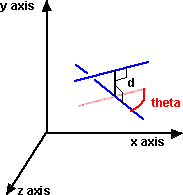# Maths - Dual Numbers

## Prerequisites

If you are not familiar with this subject you may like to look at the following pages first:

## Description

Again dual numbers are similar to the form for complex numbers above except that:

w2 = 0

Algebras which have this property are known as grassmann Algebras, in Clifford Algebra terms, this is the outer or exterior product. Vector cross products also have the same property.

It may seem strange that the square root of a non-zero quantity is zero, although it makes some kind of sense, because the square of a very small quantity is a very, very small quantity. However this is a multidimensional value where multiplying various components may produce different components, for example in Clifford Algebra multiplying one vector by another produces a scalar (So the vector component of this product will be zero). Another example is the vector cross product which produces zero when a vector is multiplied with itself.

In this case we will denote w with the symbol 'ε'

As with the other algebras here, addition is defined by adding corresponding terms and the flavor of the algebra comes from the multiplication rules. If we denote the two dimensions by 1 and e then a '1' term is given by multiplying the same types together, a 'ε' term is given by multiplying different terms. There are no sign changes. So we can define the algebra by the following table:

 a*b b.1 b.ε a.1 1 ε a.ε ε 0

### Inverse of dual multiplication

dividing a by b gives:

 a.1 + ε a.ε (a.1 + ε a.ε) (b.1 - ε b.ε) a.1 * b.1 (a.ε * b.1) - (a.1 * b.ε) -------------- = --------------- * -------------- = ------------ + ε ---------------------------- b.1 + ε b.ε (b.1 + ε b.ε) (b.1 - ε b.ε) b.12 b.12

so if b.ε = 0 then we don't need to use this method and we just divide by scalar.

otherwise the real part is: (a.1 * b.1)/b.12

and the dual part is: (a.ε * b.1 - a.1 * b.ε)/b.12

so the inverse is:

(b.1 - ε b.ε)/b.12

### Modeling with Pauli Matrix

The number a + b ε

can be represented by:

 a b 0 a

### Applications

Dual numbers can represent the relationship of two lines in 3 dimensions. This is explained further here.The relationship between the lines is represented by the dual number:

theta + d ε

where:

• theta is the angle between the lines (in radians)
• d is the distance between the lines
• ε is the dual operator

if theta=0 then the lines are parallel

if d=0 then the lines intersect